# GMAT Math : Calculating the perimeter of an equilateral triangle

## Example Questions

### Example Question #1 : Calculating The Perimeter Of An Equilateral Triangle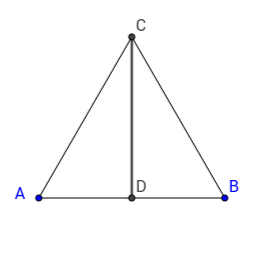The area of an equilateral triangle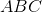is. What is the perimeter of?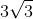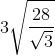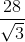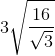Explanation:

The area is given, which will allow us to calculate the side of the triangle and hence we can also find the perimeter.

The area for an equilateral triangle is given by the formula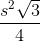, whereis the length of the side of the triangle.

Therefore,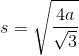, whereis the area.

Thus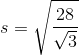, and the perimeter of an equilateral triangle is three times the side, hence, the final answer is.

### Example Question #2 : Calculating The Perimeter Of An Equilateral Triangle

A given equilateral triangle has a side length of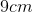. What is the perimeter of the triangle?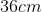Not enough information provided.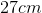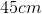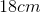Explanation:

An equilateral triangle with a side lengthhas a perimeter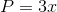.

Given: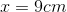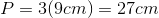### Example Question #3 : Calculating The Perimeter Of An Equilateral Triangle

A given equilateral triangle has a side length of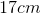. What is the perimeter of the triangle?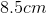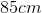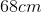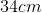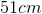Explanation:

An equilateral triangle with a side lengthhas a perimeter.

Given: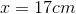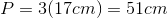### Example Question #4 : Calculating The Perimeter Of An Equilateral Triangle

A given equilateral triangle has a side length of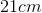. What is the perimeter of the triangle?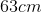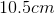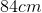Not enough information provided.Explanation:

An equilateral triangle with a side lengthhas a perimeter.

Given: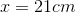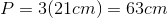### Example Question #1 : Calculating The Perimeter Of An Equilateral Triangle

Given the following regarding Triangle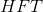.

I)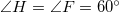II) Sideis equal tolight-years

What is the length of side?light yearslight yearslight yearslight years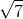light yearslight years

Explanation:

The trick to this one is to carefully put together what you are given.

We know that two of our angles are equal to 60 degrees. This means that the last angle is also 60 degrees. This make HFT an equilateral triangle.

Equilateral triangles always have equal sides and equal angles, so our last side has to be 7 light years as well.

### Example Question #6 : Calculating The Perimeter Of An Equilateral Triangle

Calculate the perimeter of an equilateral triangle whose side length is.Explanation:

The perimeter of an equilateral triangle is: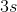Thus.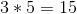### Example Question #7 : Calculating The Perimeter Of An Equilateral Triangle

Find the perimeter of an equilateral triangle whose side length is.Explanation:

To find the perimeter, you must multiply the side length by. Thus,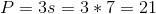### Example Question #8 : Calculating The Perimeter Of An Equilateral Triangle

Calculate the perimeter of an equilateral triangle whose side length is.Explanation:

To solve, simply multiply the side length by. Thus,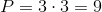Tired of practice problems?

Try live online GMAT prep today.